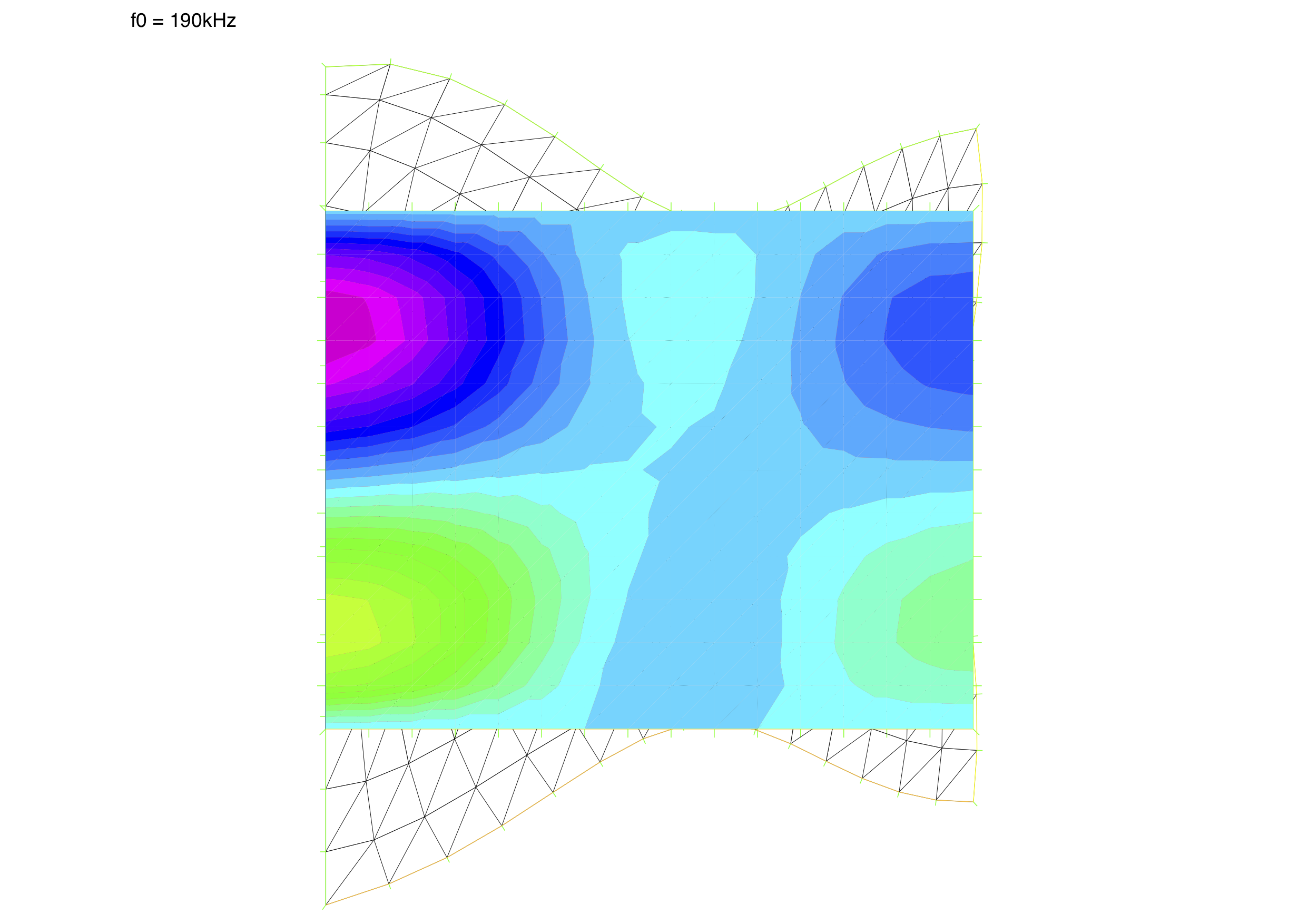# Piezoelectricity

Algorithms for solving the linear piezoelectricity equations for axisymmetric circular disc

## Problem

For time-harmonic case with stress $T$, electric displacement $D$, strain $S$ and electric field $E$, solve for displacement $u$ and potential $\phi$:

$\displaystyle{ -\omega_0^2\rho_p u_i = T_{ij,j} }$ and

$\displaystyle{ D_{i,i} = 0 }$

With:

$\displaystyle{ T_{ij} = c_{ijkl}^E S_{kl}(u) - e_{kij}E_k(\phi) }$ and

$\displaystyle{ D_{i} = e_{ikl} S_{kl}(u) +\epsilon_{ik}^S E_k(\phi) }$

where $c$ is a 3x3x3x3 elasticy tensor, $e$ - 3x3x3 piezoelectric tensor and $\epsilon$ - dielectric 3x3 matrix

## Variational form

$\displaystyle{ -\omega_0^2\int_{\Omega_p}\rho_p v_i u_i \; d\Omega = -\int_{\Omega_p} S_{i,j}(v_i) T_{ij,j} \; d\Omega }$

and

$\displaystyle{ \int_{\Omega_p} w D_{i,i} \; d\Omega = 0 }$

with $v$ and $w$ as test functions

## Algorithms

### 2D

Piezoelectricity equation on a circular disc with radius $a$ and thinkness $l$ analysed in half of its cross-section using cylindrical coordinates.

Deformation warped by a factor 100000## Authors

Author: Marek Moszyński